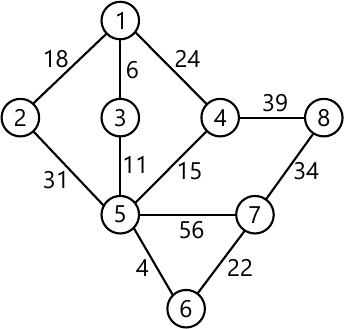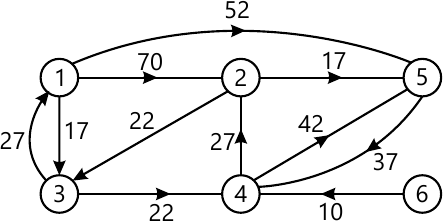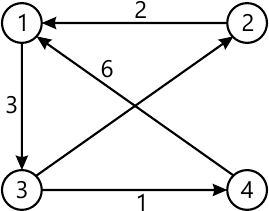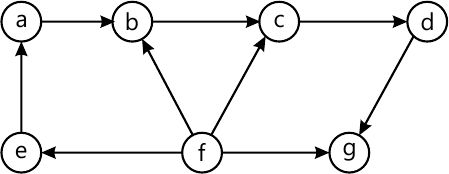MORE IN Design and Analysis of Algorithms
VTU Computer Science (Semester 4)
Design and Analysis of Algorithms
June 2012
Total marks: --
Total time: --
INSTRUCTIONS
(1) Assume appropriate data and state your reasons
(2) Marks are given to the right of every question
(3) Draw neat diagrams wherever necessary

1 (a) If t1(n) ∈ 0 (g1 (n)) and t2 (n) ∈ 0 (g2 (n)), prove that t1 (n)+ t2 (n) ∈ 0 (max {g1 (n), g2 (n)}).
6 M
1 (b) If M(n) denotes the number of moves in tower of Hanoi puzzle when a disks are involved, give a recurrence relation for M(n) and solve this recurrence relation.
7 M
1 (c) Give an algorithm for selection sort. If C(n) denotes the number of times the algorithm is executed (n denotes input size), obtain an expression for C(n).
7 M

2(a) Assuming that n is a power of 2, solve the recurrence relation T(n)=2T (n/2) +2. Take T(2)=1 and T(1)=0.
5 M
2(b) If n∈[2k-1, 2k], prove that binary search algorithm makes at most K element comparisons for a successful search and either K-1 or K comparisons for an unsuccessful search.
6 M
2(c) Give an algorithm for merge sort.
5 M
2(d) Consider the numbers given below. Show how partitioning algorithm of quick sort will place 106 in its correct position. Show all the steps clearly.
 106 117 128 134 141 91 84 63 42
4 M

3 (a) Let J be a set of K jobs and σ= i1, i2, i3,........, ik be a permutation of jobs in J such that di1 ≤ di2 ≤ ......... ≤ dik. Prove that J is a feasible solution if and only if the jobs in J can be processed in the order σ without violating any deadline.
7 M
3 (b) Using Prim's algorithm, determine minimum cost spanning tree for the weighted graph shown below, fig. Q3(b):7 M
3 (c) In the weighted diagram given below, fig. Q3(c) determine the shortest paths from vertex 1 to all other vertices.6 M

4 (a) Obtain the shortest paths from every vertex to every other vertex in the diagram given below: fig Q4(a)10 M
4 (b) Using Warshall's algorithm, obtain the transitive closure of the matrix given below: $R=\begin{pmatrix} 0 &1 &0 &0 \\0 &0 &0 &1 \\0 &0 &0 &0 \\ 1&0 &1 &0 \end{pmatrix}$
10 M

5 (a) Show how insertion sort algorithm arranges the following members in increasing order.
 61 28 9 85 34
6 M
5 (b) Obtain topological sorting for the diagram given below:6 M
5 (c) Given algorithm for the following:
i) Comparison counting: ii) Distribution counting.
8 M

6 (a) Define the following: i) Tractable problems; ii) Class P; iii) Class NP; iv) Polynomial reduction; v) NP complete problems.
5 M
6 (b) State subset sum problem. Using back tracking, obtain a solution to the subset sum problem by taking s={6, 8, 2, 14} and d=16.
7 M
6 (c) Explain approximation algorithms for NP - hard problems in general. Also discuss approximation algorithms for knapsack problem.
8 M

7 (a) What is prefix computation problem? Give the algorithm for prefix computation which uses
i) n processors; ii) n/log n, processors. Obtain the time complexities of these algorithms.
10 M
7 (b) For an n×n matrix M with non negative integer coefficients, define M and give an algorithm for computing M. Prove that M can be computed from an n×n matrix M in 0(log n) time using n3+∈ common CRCW PRAM processors for any fixed ∈>0.
10 M

Write short note on:
8 (a) Travelling salesperson problem.
5 M
8 (b) Input enhancement in string matching.
5 M
8 (c) Decision trees.
5 M
8 (d) Challenges of numerical algorithms.
5 M

More question papers from Design and Analysis of Algorithms